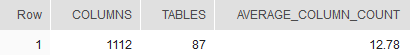## Average number of columns per table in Snowflake

Article for:

Query below returns the average number of columns per table in a database.

## Query

``````select columns,
tables,
cast(1.0 * columns / tables as decimal (14,2)) as average_column_count
from
(select count(*) as columns,
count(distinct t.table_schema || '.' ||  t.table_name) as tables
from information_schema.tables t
inner join information_schema.columns c on
c.table_schema = t.table_schema
and c.table_name = t.table_name
where t.table_type = 'BASE TABLE'
);
``````

## Columns

• columns - total number of columns in a database
• tables - number of tables in a database
• average_column_count - average number of columns in a table in a database

## Rows

• Query returns just one row

## Sample results